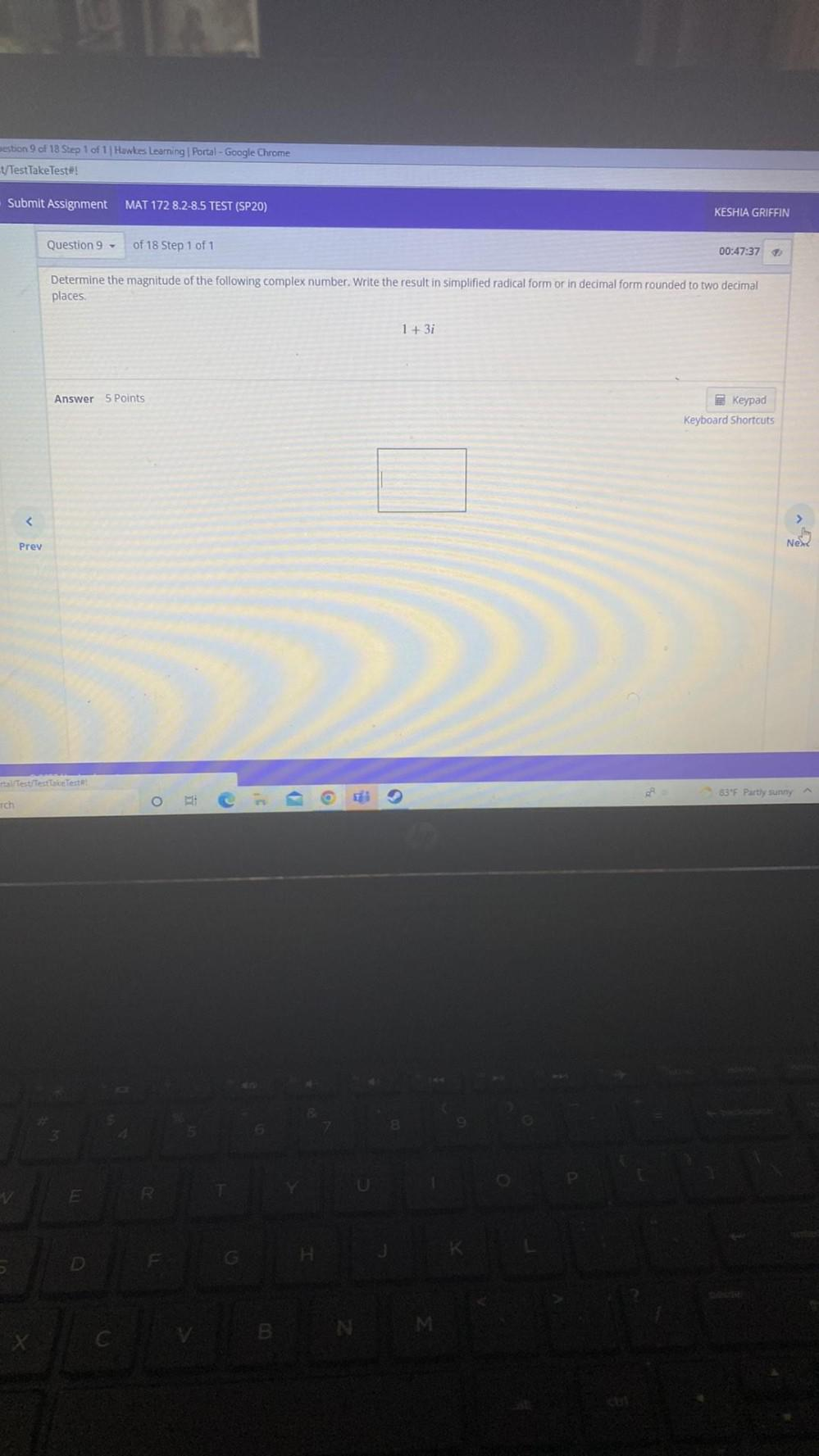Question:

# Determine the magnitude of the following complex number. Write the result in simplified radical form or in decimal form rounded to two decimal places. 1 + 3iDetermine the magnitude of the following complex number. Write the result in simplified radical form or in decimal form rounded to two decimal places. 1 + 3i